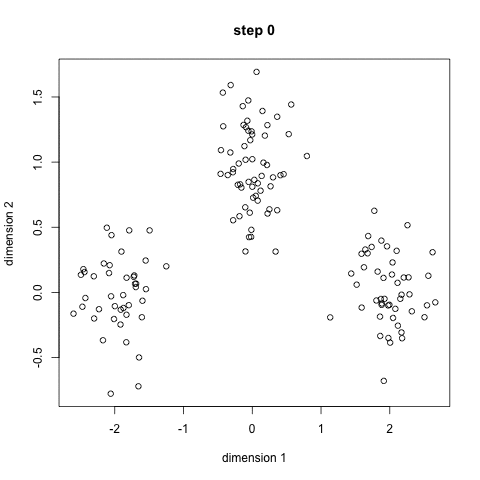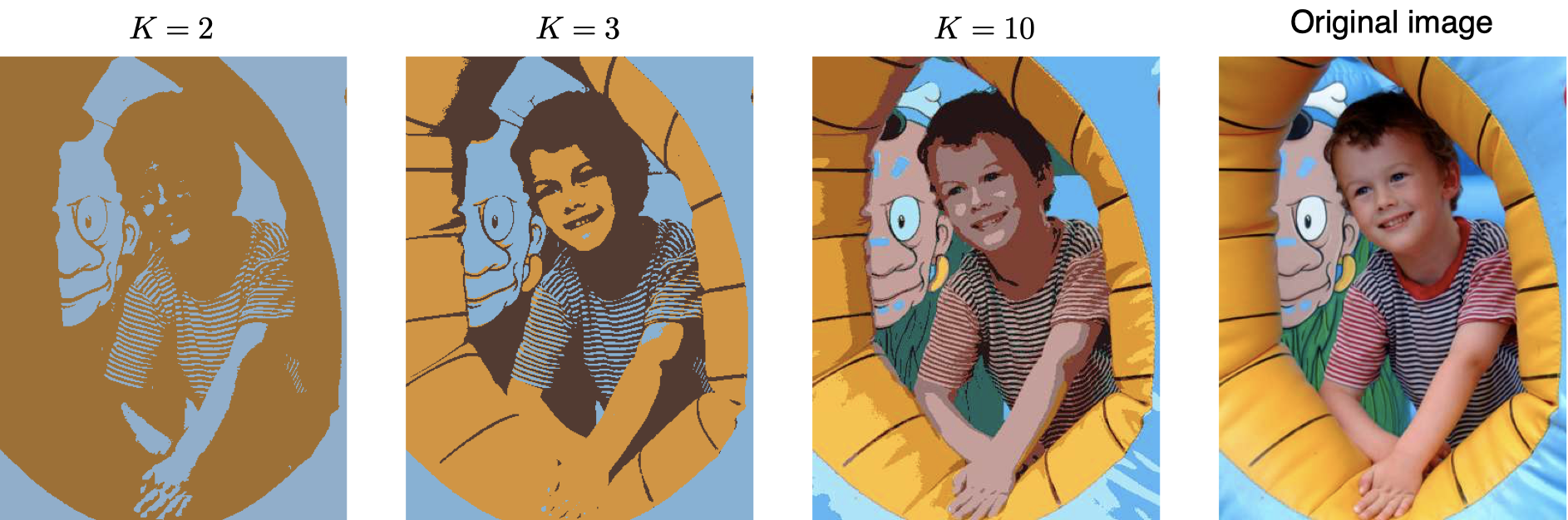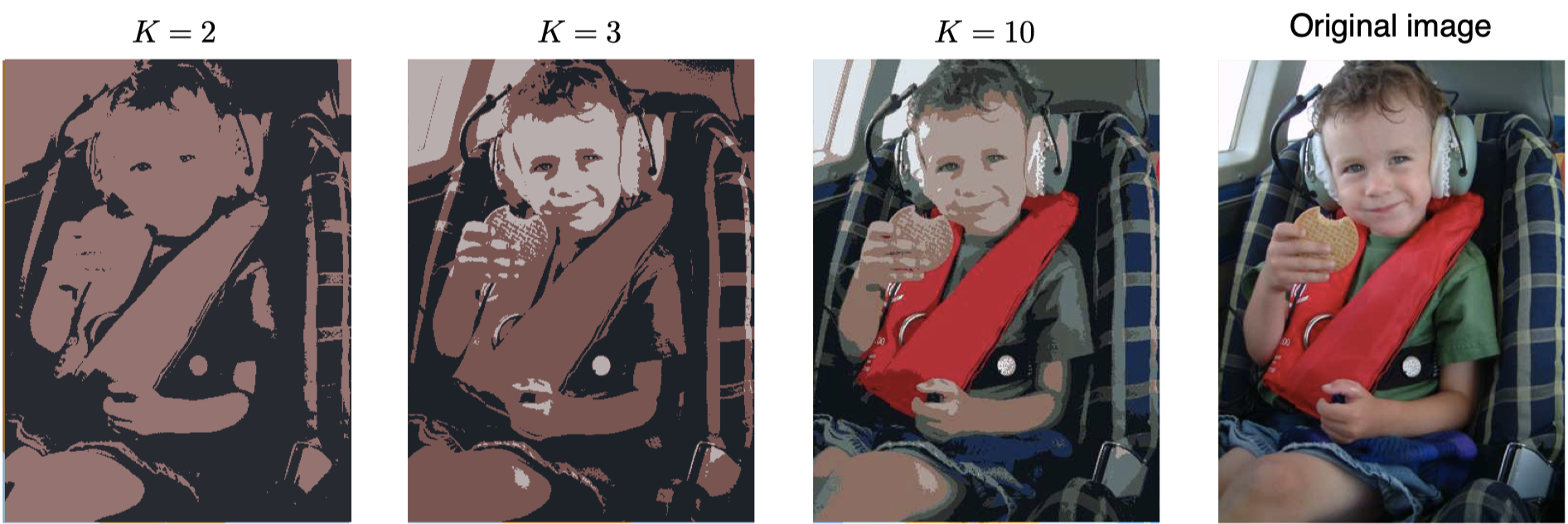K-means clustering is a type of unsupervised learning, which is used for unlabeled data (i.e., data without defined categories or groups). The goal of this algorithm is to find groups in the data, with the number of groups represented by the variable K (defined manually as an input). The algorithm works iteratively as following:

1. randomly assign K prototype vectors to the axis
2. for each data point $x_i, i \in\{1, \ldots, s\}$:
• find its nearest centroid (i.e. prototype vector)
• $$[\widehat{c_{j}}|x_{i}]=\arg \min _{j} Dist\left(x_{i}, c_{j}\right)$$
• assign $x_i$ to this cluster j
3. for each cluster j = 1...K:
• recompute its centoid $c_j$ = mean of all data points assigned to it previously
• $$c_{j}(a)=\frac{1}{n}\sum x_{i}(a) \quad \text { for } a=1 \ldots d$$ ($a$ is a particular attribute value. It can only take numerical values, since here we are computing the average. It cannot be categorical or ordinal values.)
4. stop when none of the cluster assignments change (i.e. no data points change cluster memerships anymore)Compare to all other clustering algorithms, K-means is blazingly fast. The computation required for K-mean clustering can be illustrated as:

$$iterations \times clusters(K) \times instances(x_i) \times dimensions$$

## Properties of K-means

• There are always K clusters.
• There is always at least one item in each cluster.
• The clusters are non-hierarchical and they do not overlap.
• Every member of a cluster is closer to its cluster than any other cluster

## Some Features of K-means

The variable K indicates the number of groups is predetermined manually, also called a hyper-parameter. Hence, it could be helpful to do feature analysis prior to decide what is the K.

The position of starting prototype vectors are chosen randomly. The algorithm will converge slower if they are close to each other. There are ways to optimize it, such as K-means++.

### K-means++

K-means++ is a strategy to choose the initial centroids:

1. randomly choose one initial centroid $c_1$
2. for all data points, calculate the distance between them and $c_1$
3. choose a new centroid: the longer the distance calculated in the previous step, the higher the chance this point is selected as the new centroid
4. repeat step 2 and 3 until number of prototype vectors (K) are picked
5. then do the standard K-means iteration algorithm

## K-means Implementation

### Image Compression